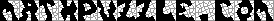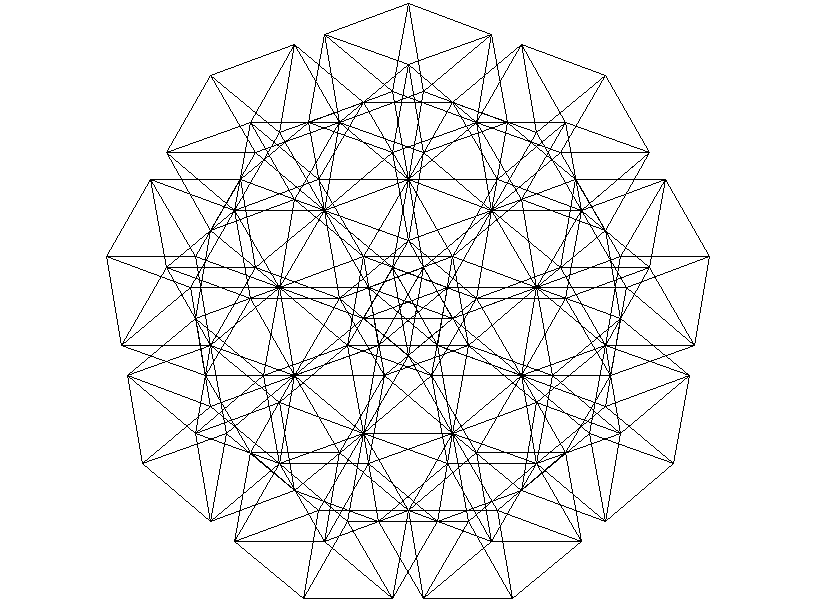This header plots the critical line of the Riemann Zeta Function.  A complete understanding wins a \$1,000,000 prize.
 . . .Main Links Orders Post Next Page Next + 10

The Chromatic Number of the Plane
I made a discovery relating to this topic -- a new coloring of the plane in seven colors.What is the minimum number of colors needed to color the plane so that no two points of the same color are exact one unit apart?  If you look at the figure above, you can see that the answer is at most seven colors.  A few unit circles have been included on the diagram.  If you look at the widget below, you'll see that answer is four colors or more.  Just toss it onto the plane.  It's not hard to prove that at least four colors are necessary.A widget that shows that the chromatic number of the plane is at least 4.
Thus, we know that the chromatic number of the plane is either 4, 5, 6, or 7 at this point.  It's still an open question.  See Graph Theory Open Problems, Mathematical Coloring Book, and Geometry Junkyard references for a little more on the history of this problem.  Alex Soifer says his Mathematical Coloring Book will be finished by the end of 1999, which isn't far off.  One object mathematicians desire is a unit graph that requires five colors.  So far, no-one has found one.  For fun, perhaps you'd enjoy finding a four-coloring for the following unit graph.Is this graph four-colorable?